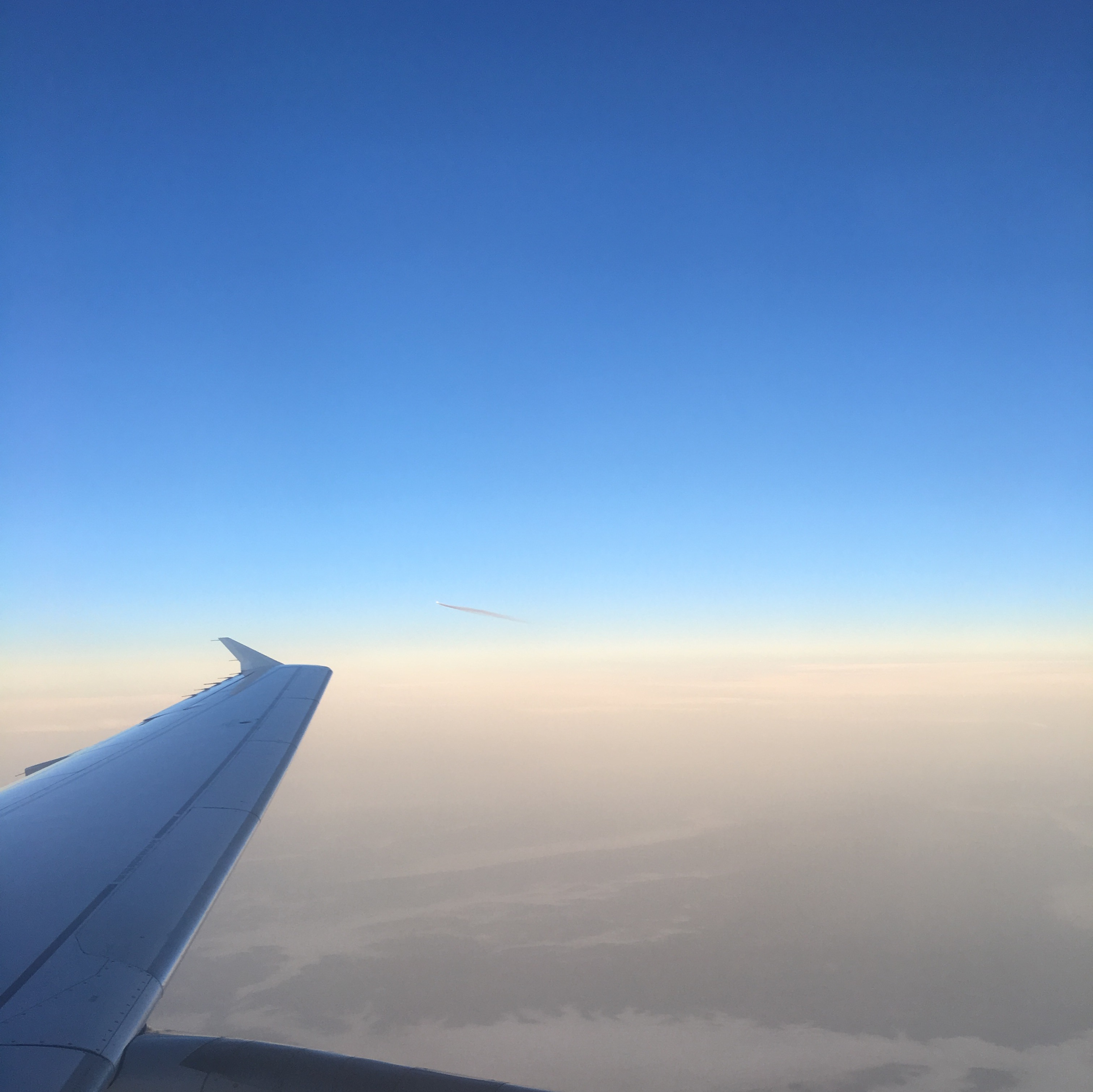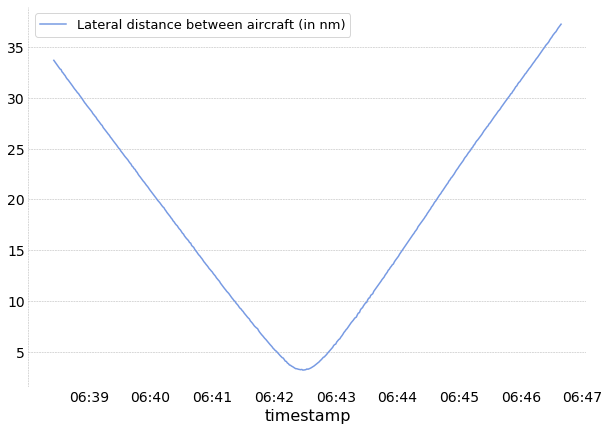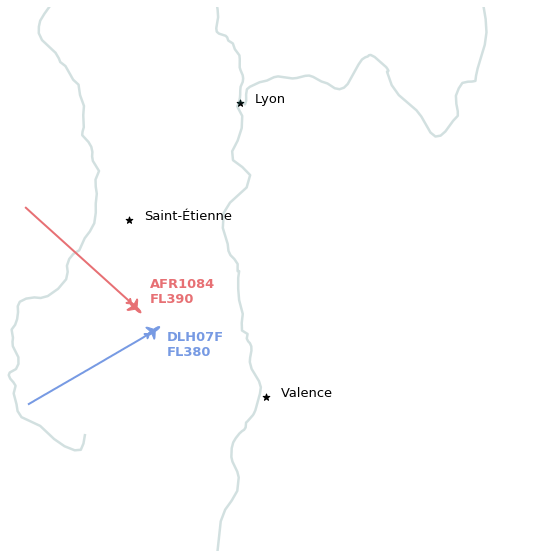# Is this a plane?¶On my way to the Opensky Network workshop 2018, I could catch a picture of this aircraft which looked really close. I wondered how close it came to us considering that the usual separation rule between aircraft is of 5 nautical miles (horizontal) and 1000ft (vertical).

First let’s get information about the flight I was on.

```from traffic.data import opensky

flight = opensky.history(
"2018-11-15 06:00",  # UTC
"2018-11-15 08:00",
callsign='DLH07F', # of course, you need to know the callsign of your flight
return_flight=True
)
```

I can check the shape of the trajectory with a good overview of Frankfurt approach up to the North.

Flight DLH07F
• aircraft: 3c6608 / D-AIPH (A320)
• origin: 2018-11-15 07:16:18
• destination: 2018-11-15 08:58:38

The picture is timestamped at 06:42 UTC. Let’s have a look of what came around.

```from datetime import timedelta

p = flight.at("2018-11-15 06:42")

around = opensky.history(
p.name - timedelta(minutes=5),
p.name + timedelta(minutes=5),
bounds=(
p.longitude - 0.5, p.latitude - 0.5,
p.longitude + 0.5, p.latitude + 0.5
)
)
around
```
Traffic with 10 identifiers
count
icao24 callsign
3944ec AFR1084 496
3c6608 DLH07F 430
406440 EZY201G 238
4ca816 RYR27NB 227
406229 EZY71XZ 186
495292 TAP1245 184
4ca813 RYR63TL 181
392ae4 HOP11JK 133
461fa5 FIN4YC 45
4ca80f RYR49ME 9

It seems we get a lot of messages of this AFR1084 flight (Paris to Tunis). Let’s plot their lateral distance vs. time.

```%matplotlib inline
import matplotlib.pyplot as plt

with plt.style.context('traffic'):

fig, ax = plt.subplots(figsize=(10, 7))

flight.distance(around['AFR1084']).plot(
ax=ax, x='timestamp', y='lateral',
label="Lateral distance between aircraft (in nm)"
)
```Wow, less than 5nm. We came really close! We can now plot a map, with information confirming we were properly separated (1000ft). That is only 300m of altitude difference: it really felt we were flying the same altitude though.

```from cartes.crs import Lambert93
from cartes.osm import Nominatim
from traffic.drawing import countries, rivers
from traffic.drawing.markers import rotate_marker, aircraft

with plt.style.context("traffic"):

fig, ax = plt.subplots(subplot_kw=dict(projection=Lambert93()))

ax.set_extent((3.9, 6, 44.5, 46))

Nominatim.search("Lyon").point.plot(
ax, s=50, marker="*", text_kw=dict(s="Lyon")
)
Nominatim.search("Valence, France").point.plot(
ax, s=50, marker="*", text_kw=dict(s="Valence")
)
Nominatim.search("Saint-Étienne").point.plot(
ax, s=50, marker="*", text_kw=dict(s="Saint-Étienne")
)

for i, cs in enumerate(["DLH07F", "AFR1084"]):
x, *_ = (
around[cs].before("2018-11-15 06:42").last(minutes=5).plot(ax, linewidth=2)
)

p = around[cs].at("2018-11-15 06:42")
p.plot(
ax,
s=300,
marker=rotate_marker(aircraft, p.track),
color=x.get_color(),
text_kw=dict(
s=f"{cs}\nFL{p.altitude/100:.0f}",
verticalalignment="top" if i % 2 == 0 else "bottom",
color=x.get_color(),
fontweight="bold",
),
)

ax.spines['geo'].set_visible(False)
```